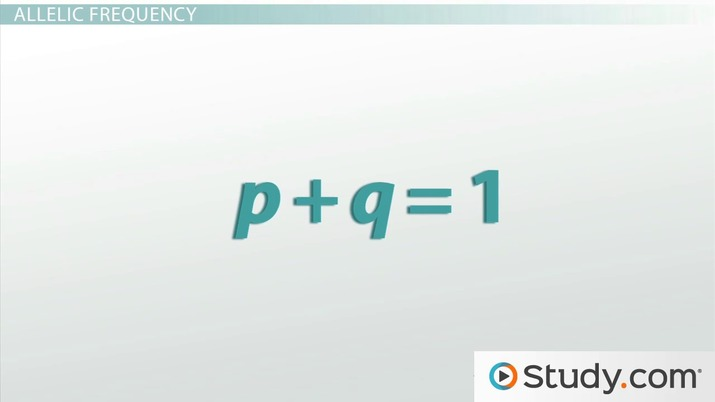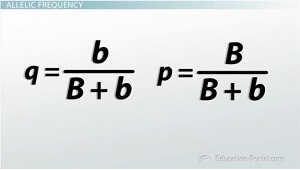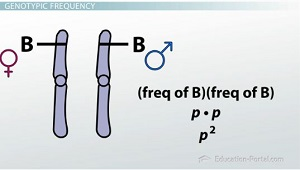Science Courses / Course / Chapter

# Hardy-Weinberg Equilibrium II: The Equation

Lesson Transcript
Instructor: Greg Chin
The Hardy-Weinberg equilibrium equation helps in analyzing and understanding the gene pool of a population. Learn about this equation, including the variables it represents and where to find these variables, understand alleles and genotypes, and explore the significance of allelic and genotypic frequencies. Updated: 08/18/2021

## Conditions for Hardy-Weinberg Equilibrium

I think our research project is going to be great. I mean, think about it - we have all these great flying hamster traits we've been studying with Adrian. It's going to be really interesting to see how these traits affect the flying hamster populations. Do the coat color, tail color or fire-breath traits improve or hinder the ability of the hamsters to survive and mate?

Recall that flying hamsters are diploid, meaning they have two homologous copies of each gene. We've identified two versions, or alleles, of each gene we're going to study. That means that three different genotypes are possible in any flying hamster population. For instance, at the coat color gene, hamsters can be BB, Bb, or bb. To make our research project easier, let's also only consider traits where the distribution in males and females is equal and generations don't overlap. Hardy-Weinberg equilibrium can be calculated for genes with more than two alleles and where the generations overlap, but the math is much more complicated.Speaking of math, let's see what the Hardy-Weinberg equilibrium equation looks like: (p2 + 2pq + q2 = 1). Oh, boy. It's a polynomial equation. Well, let's push up our sleeves, take a deep breath and see if we can figure out how all these crazy letters are supposed to describe genotypic frequency.An error occurred trying to load this video.

Try refreshing the page, or contact customer support.

Coming up next: Hardy-Weinberg Equilibrium I: Overview

### You're on a roll. Keep up the good work!

Replay
Your next lesson will play in 10 seconds
• 0:06 Hardy-Weinberg Conditions
• 1:34 Allelic Frequency
• 6:00 Genotypic Frequency
• 8:38 Using the Equation
• 10:17 Summary
Save Timeline
Autoplay
Autoplay
Speed Speed

## Allelic Frequency

I think the first thing to try to understand, especially when dealing with a science equation, is the variables. At least the Hardy-Weinberg equation only has two variables, p and q. These stand for the allelic frequency of the two possible alleles for the gene in question.

Let's analyze the coat color gene in the context of the Hardy-Weinberg equation. We can arbitrarily assign p to the dominant brown allele (B) and q to the recessive white allele (b). In this example, our gene pool consists of the B and b alleles, and p and q represent the frequency at which those alleles appear in the population. But, how do we calculate allele frequency?

Allele frequency is basically the proportion the allele occupies in the gene pool. The sum of all of the alleles found in a population is known as the gene pool. To calculate p, we simply need to divide the number of B alleles by the sum of all of the alleles associated with the gene. That is B and b. Similarly, q can be calculated by dividing the number of b alleles by the sum of B + b. But, how can we determine how many B and b alleles are in a population?Well, we should be able to infer the number of B and b alleles based on the genotype of the organisms in the population, right? Let's see if that works.

Let's examine the coat color gene in a population of 1,000 hamsters. A homozygous dominant brown hamster has two B alleles, so if we saw 360 of them, that would account for 720 B alleles. A heterozygous brown hamster has one B and one b allele, so if we saw 480 of them, that would translate to 480 B and 480 b alleles. A homozygous recessive white hamster has two b alleles, so if we saw 160 white hamsters, they'd contribute 320 b alleles to our calculations. The white hamsters are obviously pretty easy to identify, but the brown ones are a little trickier since some are homozygous and some are heterozygous. Luckily, we can distinguish the homozygotes from the heterozygotes if we genetically type the brown hamsters with a method like DNA sequencing.

Alright, let's plug all those numbers into a frequency formula. The frequency of B is equal to p, and that is equal to 720 + 480 and all that is divided by the gene pool (the sum of) 720 + 480 + 480 + 320. All of that comes out to 0.6. That means that 60% of the alleles in the gene pool are B.

Now that we've got that calculation under our belt, calculating the frequency of b should be a little bit easier now, right? The frequency of b is equal to q, and that's equal to the sum of 480 + 320 divided by the sum of 720 + 480 + 480 + 320. That's all equal to 0.4. That means that 40% of the alleles in the gene pool are b.

Note that the sum of the frequencies of the B and b alleles is one. That makes sense because together they make up 100% of the alleles in the gene pool. Let's restate the relationship between the two frequencies as a new equation that we can use in our population genetic studies, we can say that p + q = 1.

## Genotypic Frequency

Well, that's all fine and dandy, but when you're studying a population of organisms, you're interested in the organisms. The Hardy-Weinberg equation describes the ratio of different genotypes in a population. Before we tackle genotypic frequencies, let's consider a simpler example first.

If we flip two coins, what are the odds that both come up heads? There's a one-in-two chance to get heads, so that means there's a one-in-four chance of getting heads twice in a row. Since flying hamsters are diploid, a hamster with a BB genotype got one B allele from its mom and one B allele from its dad. Just like coin flipping, the chance of getting two B alleles is merely the chance of getting one B allele squared (Bˆ2). Since we know the frequency of the B allele in the gene pool is p, the chance of getting a homozygous dominant individual is p2.To unlock this lesson you must be a Study.com Member.

### Register to view this lesson

Are you a student or a teacher?

Back

### Resources created by teachers for teachers

Over 30,000 video lessons & teaching resources‐all in one place.Video lessonsQuizzes & WorksheetsClassroom IntegrationLesson Plans

I would definitely recommend Study.com to my colleagues. It’s like a teacher waved a magic wand and did the work for me. I feel like it’s a lifeline.

Jennifer B.
TeacherCreate an account to start this course today
Used by over 30 million students worldwide